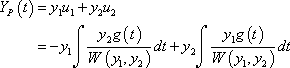# Variation of parameters to obtain PS of 2nd Order non-hom equation

The question I'm trying to solve is:

y" - 6y' + 9y = $\frac{exp(3x)}{(1+x)}$

I formulated the Gen solution which are:

y1(x) = exp(3x) and y2(x) = xexp(3x)

I've then calculated the wronskian to get: exp(6x)

I then went onto to use the variation of parameters formula, which is where I got a bit stuckI ended up with

-exp(3x)*(x - ln(x+1) + xexp(3x)*ln(1+x)

The problem is, it just doesn't look right.

I would appreciate some guidance with this problem